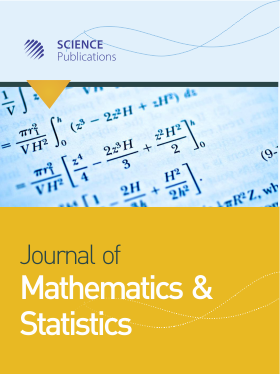Frequency: Continuous
ISSN: 1549-3644 (Print)
ISSN: 1558-6359 (Online)
Research Article Open Access

# Finding the Envelope and Efficient Frontier of Financial Assets

Farida Kachapova1 and Ilias Kachapov2
• 1 Auckland University of Technology, New Zealand
• 2 University of Auckland, New Zealand

## Abstract

Problem statement: One of the problems considered in financial mathematics is finding portfolios of given financial assets that minimize risk for targeted returns. The set of such portfolios is called the envelope of the assets. Traditionally this problem is solved as a calculus minimization problem involving partial derivatives and Lagrange multipliers. Approach: In this study we describe an invariant geometric solution that uses orthogonal projection in Euclidean space of random variables. Results: The method is applied to find the efficient portfolio and feasible region of the assets and to investigate the mean-variance relation for envelope portfolios. In particular, it is shown that the graph of this relation is the right branch of a hyperbola. The method is illustrated by an example with four financial assets. Conclusion/Recommendation: The described geometric approach can help to improve the teaching of portfolio analysis by making the concept of envelope clearer and by simplifying proofs.

Journal of Mathematics and Statistics
Volume 8 No. 3, 2012, 323-329

DOI:

Submitted On: 5 June 2012 Published On: 16 July 2012

How to Cite: Kachapova, F. & Kachapov, I. (2012). Finding the Envelope and Efficient Frontier of Financial Assets. Journal of Mathematics and Statistics, 8(3), 323-329. https://doi.org/10.3844/jmssp.2012.323.329

• 4,064 Views